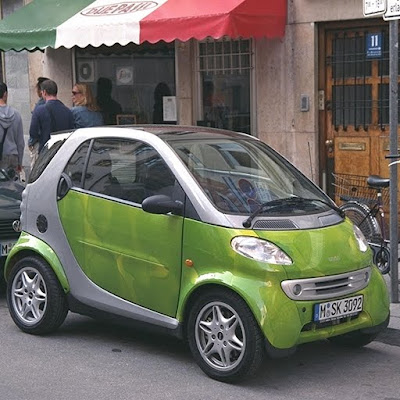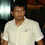## Monday, June 14, 2010

Pixelation is process when pixel at x,y is duplicated into x+dx,y+dy rectangle. Pixelation GLSL fragment code:

`uniform sampler2D tex;void main(){ float dx = 15.*(1./512.); float dy = 10.*(1./512.); vec2 coord = vec2(dx*floor(gl_TexCoord.x/dx),                   dy*floor(gl_TexCoord.y/dy)); gl_FragColor = texture2D(tex, coord);}`

Smartand pixelated version of it1.Hi,

Can we achieve this pixelation in hexagonal pixels ? If yes , can you please guide me on how to achieve this.?

1.You can indeed make pixelation into hexagonal pixels.
By using this article on hexagonal grids - one can write hexagonal pixelation GLSL fragment shader as:
_______________________________________________
#version 130

uniform sampler2D Texture0;

#define H 0.02
#define S ((3./2.) * H/sqrt(3.))

vec2 hexCoord(ivec2 hexIndex) {
int i = hexIndex.x;
int j = hexIndex.y;
vec2 r;
r.x = i * S;
r.y = j * H + (i%2) * H/2.;
return r;
}

ivec2 hexIndex(vec2 coord) {
ivec2 r;
float x = coord.x;
float y = coord.y;
int it = int(floor(x/S));
float yts = y - float(it%2) * H/2.;
int jt = int(floor((1./H) * yts));
float xt = x - it * S;
float yt = yts - jt * H;
int deltaj = (yt > H/2.)? 1:0;
float fcond = S * (2./3.) * abs(0.5 - yt/H);

if (xt > fcond) {
r.x = it;
r.y = jt;
}
else {
r.x = it - 1;
r.y = jt - (r.x%2) + deltaj;
}

return r;
}

void main(void)
{
vec2 xy = gl_TexCoord.xy;
ivec2 hexIx = hexIndex(xy);
vec2 hexXy = hexCoord(hexIx);
vec4 fcol = texture2D(Texture0, hexXy);
gl_FragColor = fcol;
}
____________________________________________
Good luck!

2.Hi,

Thank you for the reply. I think i have achieved it.
Thank you.

3.Hi,

I tried the hexagonal pixelation from your code, I can see hexagons, but the sides of hexagons are not smooth, they look distorted. etc. Can you help me to sort it out?

4.Well, I can only guess. It may be because of "un-smooth" behavior of function hexIndex() which clusters pixels into hexagons. Possibly problem are with pixels which are on boundary between two hexagons, so problem arises in that case - to which hexagon we should attach pixel ? So we must somehow deal with this edge case. Another thing that can be that some hexagon edge lines is at 60 degrees between edge and horizontal and/or vertical and because in reality monitor has some aspect ratio (that is real texture texels are not really squares, but rectangles instead) - you may get such total effect. And other reasons may apply - such as small accuracy of GLSL functions , integer rounding errors, bugs in different video card manufacturer drivers and etc ... You can also try to implement line anti-aliasing effect with GLSL, because with anti-aliasing enabled hexagon edges may look nicer.

So you must experiment yourself and choose such concrete implementation which fits your current needs. I just showed main principle here.

Good luck !

5.Hi,

Thank you for the help. Appreciate it.

Comment will be posted after comment moderation.